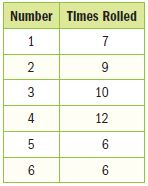Homework Explained - Math Practice 101Dear guest, you are not a registered member. As a guest, you only have read-only access to our books, tests and other practice materials.

As a registered member you can:

Registration is free and doesn't require any type of payment information. Click here to Register.
Go to page:
Chapter 9: Probability; Chapter Review

### Problem Solving

For Exercises 1 and 2, a number cube is rolled. The table shows the results.• Question 1

What is the experimental probability that the next roll will be four?

• P(4)=
• Question 2

How does the experimental probability of rolling 4 compare to the theoretical probability? Provide a reason for any discrepancy.

• Type below:
• Question 3

Ginger and Micah are playing a game in which a coin is tossed twice. If heads comes up exactly once, Ginger wins. Otherwise, Micah wins. Find the sample space using a  tree diagram. Then find the probability that Ginger wins.

• Type below:
• Question 4

In how many ways can the picture frames shown be arranged in a line on the wall?• Question 5

A bag of animal crackers contains 6 monkeys, 5 giraffes, 6 elephants, and 3 tigers. Maria selects a cracker at random. Then Bryan selects a cracker at random. What is the probability that two tigers are chosen?

Yes, email page to my online tutor. (if you didn't add a tutor yet, you can add one here)